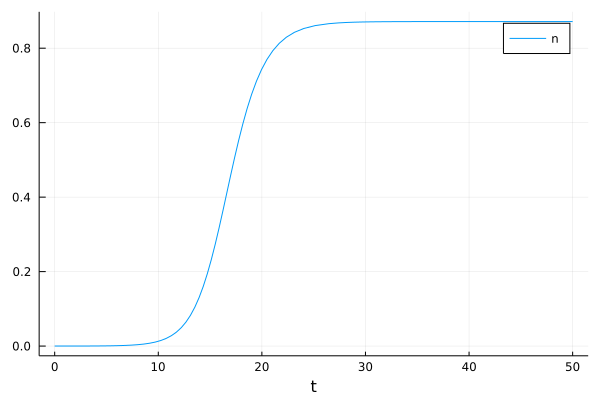## QuantumCumulants.jl

Generalized mean-field equations in open quantum systems
Author qojulia
Popularity
27 Stars
Updated Last
2 Years Ago
Started In
February 2020

# QuantumCumulants.jl

QuantumCumulants.jl is a package for the symbolic derivation of mean-field equations for quantum mechanical operators in Julia. The equations are derived using fundamental commutation relations of operators. When averaging these equations they can be automatically expanded in terms of cumulants to an arbitrary order (generalized mean-field approximation). This results in a closed set of symbolic differential equations, which can also be solved numerically.

For the application of commutation relations QuantumCumulants.jl implements a simple noncommutative algebra, where any commutation relations are applied immediately. All other symbolic simplification and rewriting is done using the Symbolics.jl package.

To obtain a numerical solution, equations derived with QuantumCumulants.jl can be converted to ModelingToolkit.jl and subsequently solved with DifferentialEquations.jl.

## Development status

Note that QuantumCumulants.jl is still at an early stage of development.

## Installation

The package can be installed with

`|pkg> add QuantumCumulants`

## Documentation

Please refer to the latest Documentation for more details and examples.

## Short example

To briefly illustrate how QuantumCumulants.jl works, here's how you can implement a first-order mean-field model of a laser with a single atom as a gain medium:

```using QuantumCumulants

h_cav = FockSpace(:cavity)
h_atom = NLevelSpace(:atom, (:g,:e))
h = tensor(h_cav, h_atom)

@cnumbers Δ g κ γ ν
@qnumbers a::Destroy(h) σ::Transition(h)

H = Δ*a'*a + g*(a'*σ(:g,:e) + a*σ(:e,:g))
J = [a,σ(:g,:e),σ(:e,:g)]
rates = [κ,γ,ν]

eqs = meanfield([a,σ(:g,:e),σ(:e,:e)], H, J; rates=rates, order=1)

using ModelingToolkit, OrdinaryDiffEq
sys = ODESystem(eqs)
p0 = (Δ=>0, g=>1.5, κ=>1, γ=>0.25, ν=>4)
u0 = ComplexF64[1e-2, 0, 0]
prob = ODEProblem(sys,u0,(0.0,50.0),p0)
sol = solve(prob,RK4())

using Plots
n = abs2.(sol[a])
plot(sol.t, n, xlabel="t", label="n")```The above code implements the Jaynes-Cummings Hamiltonian describing an optical cavity mode that couples to a two-level atom. Additionally, the decay processes are specified. Then, mean-field equations for the average values of the operators `[a,σ(:g,:e),σ(:e,:e)]` are derived and expanded to first order (average values of products are factorized). For the numerical solution an `ODESystem` (from ModelingToolkit.jl) is created and solved with the OrdinaryDiffEq.jl library. Finally, the time dynamics of the photon number `n` is plotted.

## Citing

If you find QuantumCumulants.jl useful in your research, please consider citing this paper:

``````@article{plankensteiner2021quantumcumulants,
title={{QuantumCumulants.jl}: {A Julia} framework for generalized mean-field equations in open quantum systems},
author={Plankensteiner, David and Hotter, Christoph and Ritsch, Helmut},
journal={arXiv preprint arXiv:2105.01657},
year={2021},
url={https://arxiv.org/abs/2105.01657}
}
``````

### Required Packages

View all packages

### Used By Packages

No packages found.